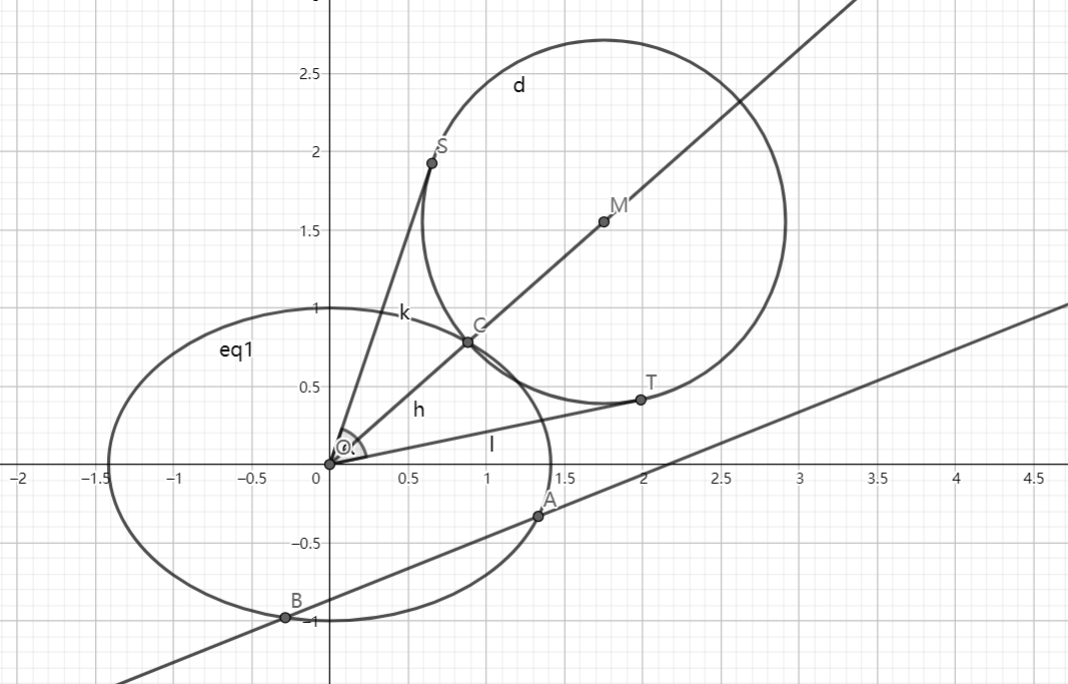# SAT1000 - P843

Geometry Level pendingAs shown above, the ellipse has equation: $\dfrac{x^2}{2}+y^2=1$, line $l: y=k_1 x-\dfrac{\sqrt{3}}{2}$ intersects with the ellipse at point $A,B$.

Point $C$ is on the ellipse and line $OC$ has slope $k_2$, $k_1 k_2=\dfrac{\sqrt{2}}{4}$.

$M$ is a point on ray $OC$, $|MC| : |AB| = 2 : 3$, and the radius of circle $M$ is $|MC|$, $OS,OT$ are two tangent lines of circle $M$ and $S,T$ are tangent points.

Then find the maximum value of $\angle SOT$ (in radians), and find the slope of $l$ when $\angle SOT$ reaches the maximum.

Let $\theta$ be the maximum value of $\angle SOT$ (in radians), $k$ is the slope of $l$. Submit $\lfloor 1000(\theta+|k|)\rfloor$.

Have a look at my problem set: SAT 1000 problems

×